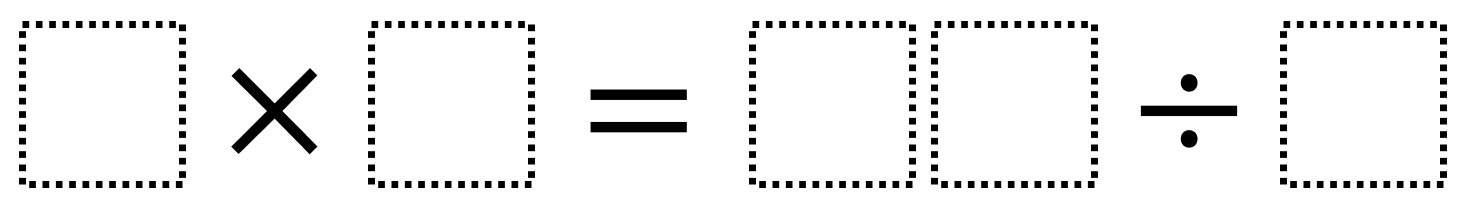# Multiply and Divide Within A Hundred 2

Directions: Using the digits 2 to 9 at most one time each, place a digit in each box to make a correct equations where the value is as close to 38 as possible.### Hint

What digits would be better worse to divide by? What are the greatest values you can make on the division side?

The closest you can get to 38 is 7 x 6 = 84 ÷ 2 (which equals 42 on both sides).

Source: Robert Kaplinsky

## Multiply and Divide Within A Hundred 1

Directions: Using the digits 2 to 9 at most one time each, place a digit …

### One comment

1.6 X 8 = 192/4